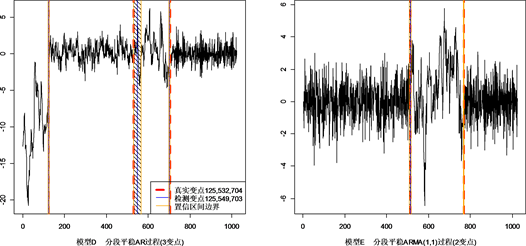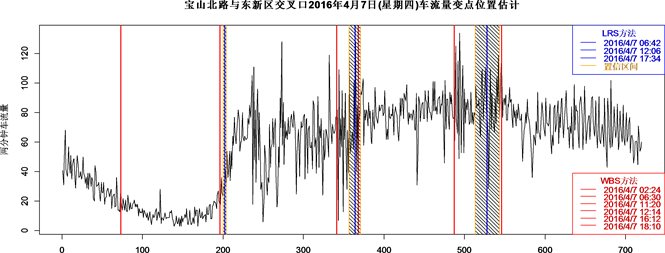﻿ 分段平稳时间序列中的多变点检测

# 分段平稳时间序列中的多变点检测Multiple Change-Points Detection of Piecewise Stationary Time Series

Abstract: The change-point problem has a wide range of applications in the industrial, financial, meteorology and other fields. A method for estimating the numbers, locations of change-point by building the Likelihood ratio scan (LRS) statistics, combined with the Minimum description length (MDL) principle has been proposed. It reduces the computationally infeasible global mul-tiple-change-point estimation problem to a number of single-change-point detection problems in various local windows by effective segmentation. In order to provide more information for describing change points, we have constructed confidence intervals for each of the change points. Finally, extensive simulation studies and example analysis of traffic show the LRS usability practice.

1. 引言

2. 模型介绍

2.1. 基本假设

${X}_{t}=g\left(\theta ,{X}_{t},{ϵ}_{t}\right)$ (1)

$\left\{{X}_{1},\cdots ,{X}_{{\tau }_{1}^{0}}\right\}\in Μ\left({\theta }_{1}^{0}\right),\text{}\left\{{X}_{{\tau }_{1}^{0}+1},\cdots ,{X}_{n}\right\}\in Μ\left({\theta }_{2}^{0}\right)$ (2)

${L}_{n}\left(\tau ,{\theta }_{1},{\theta }_{2}\right)={L}_{1n}\left(\tau ,{\theta }_{1}\right)+{L}_{2n}\left(\tau ,{\theta }_{2}\right)$

$\stackrel{^}{\tau }=\mathrm{arg}\underset{1\le \tau \le n}{\mathrm{max}}{L}_{n}\left[\tau ,{\stackrel{^}{\theta }}_{1n}\left(\tau \right),{\stackrel{^}{\theta }}_{2n}\left(\tau \right)\right]$ (3)

2.2. 变点估计的渐近分布

${W}_{\tau }=\left\{\begin{array}{l}{\sum }_{t=1}^{\tau }\left({l}_{t}\left({\theta }_{1}^{0}\right)-{l}_{t}\left({\theta }_{2}^{0}\right)\right),\text{}\tau >\text{0,}\\ 0,\text{}\tau =\text{0,}\\ {\sum }_{t=\tau }^{-1}\left({l}_{t}\left({\theta }_{2}^{0}\right)-{l}_{t}\left({\theta }_{1}^{0}\right)\right),\text{}\text{ }\text{ }\tau <\text{0}\text{.}\end{array}$ (4)

$\tau >0$${X}_{t}\in M\left({\theta }_{2}^{0}\right)$$\tau <0$${X}_{t}\in M\left({\theta }_{1}^{0}\right)$ 。参照Ling (2016)的定理2.2(b)，对于固定的 ${\theta }_{1}^{0}$${\theta }_{2}^{0}$

${\stackrel{^}{\tau }}_{1}-{\tau }_{1}^{0}\stackrel{d}{\to }\mathrm{arg}\underset{\tau \in ℤ}{\mathrm{max}}{W}_{\tau }$ (5)

${\left(\stackrel{^}{{d}^{\prime }}\stackrel{^}{\sum }\stackrel{^}{{d}^{\prime }}\right)}^{2}{\left(\stackrel{^}{{d}^{\prime }}\stackrel{^}{\Omega }\stackrel{^}{{d}^{\prime }}\right)}^{-1}\left(\underset{r\in \left[-M,M\right]}{\mathrm{arg}\mathrm{max}}{W}_{⌊\stackrel{^}{m}r⌋}\right)\stackrel{d}{\to }\underset{r\in \left[-M,M\right]}{\mathrm{arg}\mathrm{max}}\left\{B\left(r\right)-\frac{1}{2}|r|\right\}$ (6)

$|{F}_{d}\left(x\right)-P\left(\mathrm{arg}{\mathrm{max}}_{\tau \in \left[-s,s\right]}{W}_{⌊\tau ⌋}\le x\right)|\le \epsilon$

$\begin{array}{l}|{F}_{d}\left(x\right)-P\left(m\mathrm{arg}{\mathrm{max}}_{r\in \left[-M,M\right]}{W}_{⌊\stackrel{^}{m}r⌋}x\right)|\\ =|{F}_{d}\left(x\right)-P\left(\mathrm{arg}{\mathrm{max}}_{r\in \left[-mM,mM\right]}{W}_{⌊r⌋}\le x\right)|\le \epsilon \end{array}$

${F}_{d}\left(x\right)\approx P\left(\mathrm{arg}{\mathrm{max}}_{r\in R}\left[B\left(r\right)-|r|/2\right]\le x\right)$

$f\left(x\right)=\frac{3}{2}{\text{e}}^{|x|}\Phi \left(\frac{3}{2}\sqrt{|x|}\right)-\frac{1}{2}\Phi \left(\frac{\sqrt{|x|}}{2}\right)$ (7)

$CI=\left[{\stackrel{^}{\tau }}_{1}-\left[\Delta {F}_{\alpha /2}\right]-1,{\stackrel{^}{\tau }}_{1}+\left[\Delta {F}_{\alpha /2}\right]+1\right]$ (8)

3. 基于似然比扫描 (LRS)方法的多变点估计

3.1. 基本假设

3.2. 基于似然比扫描统计量的多变点检测步骤

${W}_{t}\left(h\right)=\left\{t-h+1,\cdots ,t+h\right\}$${X}_{{W}_{t}\left(h\right)}=\left\{{X}_{t-h+1},\cdots ,{X}_{t+h}\right\}$

${S}_{h}\left(t\right)=\frac{1}{h}{L}_{1h}\left(t,{\stackrel{^}{\theta }}_{1}\right)+\frac{1}{h}{L}_{2h}\left(t,{\stackrel{^}{\theta }}_{2}\right)-\frac{1}{h}{L}_{\cdot h}\left(t,\stackrel{^}{\theta }\right)$

${L}_{1h}\left(t,{\stackrel{^}{\theta }}_{1}\right)$${L}_{2h}\left(t,{\stackrel{^}{\theta }}_{2}\right)$${L}_{\cdot h}\left(t,\stackrel{^}{\theta }\right)$ 分别是相应观测序列 ${\left\{{X}_{s}\right\}}_{t-h+1,\cdots ,t}$${\left\{{X}_{s}\right\}}_{t+1,\cdots ,t+h}$${\left\{{X}_{s}\right\}}_{{W}_{t}\left(h\right)}$ 的条件似然函数。具体地说，样本 $z=\left\{{z}_{1},\cdots ,{z}_{n}\right\}$ 的条件似然函数为

$L\left(\theta \right)=\underset{t=1}{\overset{n}{\sum }}{l}_{t}\left(\theta \right)\equiv \underset{t=1}{\overset{n}{\sum }}\mathrm{log}{f}_{\theta }\left({z}_{t}|{z}_{t-1},{z}_{t-2},\cdots \right)$

${\stackrel{^}{\mathcal{J}}}^{\left(1\right)}=\left\{m\in \left\{h,h+1,\cdots ,n-h\right\}:{S}_{h}\left(m\right)=\underset{t\in \left(m-h,m+h\right)}{\mathrm{max}}{S}_{h}\left(t\right)\right\}$

$t\notin \left[h,\cdots ,\left(n-h\right)\right]$${S}_{h}\left(t\right)\triangleq 0$ 。即如果 ${S}_{h}\left(m\right)$ 是扫描窗口 $\left[m-h+1,m+h\right]$ 内的最大值，则m就是该扫描窗口的局部变点估计。记 $\stackrel{^}{m}$ 为估计变点的数目，表示  中的元素个数。

$\text{MDL}\left(m,\mathcal{J}\right)=\mathrm{log}\left(m\right)+\left(m+1\right)\mathrm{log}\left(n\right)+\underset{j=1}{\overset{m+1}{\sum }}\underset{k=1}{\overset{{c}_{j}}{\sum }}\mathrm{log}\left({\zeta }_{j,k}\right)+\underset{j=1}{\overset{m+1}{\sum }}\frac{{d}_{j}}{2}\mathrm{log}\left({n}_{j}\right)-\underset{j=1}{\overset{m+1}{\sum }}{L}_{j}\left({\stackrel{^}{\theta }}_{j};{X}_{j}\right)$

$\text{MDL}\left(m,\mathcal{J}\right)=\mathrm{log}\left(m\right)+\left(m+1\right)\mathrm{log}\left(n\right)+\underset{j=1}{\overset{m+1}{\sum }}\mathrm{log}\left({p}_{j}\right)+\underset{j=1}{\overset{m+1}{\sum }}\frac{{p}_{j}+2}{2}\mathrm{log}\left({n}_{j}\right)-\underset{j=1}{\overset{m+1}{\sum }}{L}_{j}\left({\stackrel{^}{\theta }}_{j};{X}_{j}\right)$

$\left({\stackrel{^}{m}}^{\left(2\right)},{\stackrel{^}{\mathcal{J}}}^{\left(2\right)}\right)=\underset{m=|\mathcal{J}|,\mathcal{J}\subseteq {\stackrel{^}{\mathcal{J}}}^{\left(1\right)}}{argmin}\text{MDL}\left(m,\mathcal{J}\right)$

${E}_{j}\left(h\right)=\left\{{\stackrel{^}{\tau }}_{j}^{\left(2\right)}-2h+1,\cdots ,{\stackrel{^}{\tau }}_{j}^{\left(2\right)}+2h\right\}$${X}_{{E}_{j}\left(h\right)}=\left\{{X}_{{\stackrel{^}{\tau }}_{j}^{\left(2\right)}-2h+1},\cdots ,{X}_{{\stackrel{^}{\tau }}_{j}^{\left(2\right)}+2h}\right\}$

${\stackrel{^}{\tau }}_{j}^{\left(3\right)}=\underset{\tau \in \left({\stackrel{^}{\tau }}_{j}^{\left(2\right)}-h,{\stackrel{^}{\tau }}_{j}^{\left(2\right)}+h\right]}{\mathrm{arg}\mathrm{max}}{L}_{j}\left(\tau ,{\stackrel{^}{\theta }}_{j},{\stackrel{^}{\theta }}_{j+1}\right)$

3.3. 渐近性质

$\left\{\begin{array}{l}E\left[{l}_{k}\left({\theta }_{j+1}^{0}\right)\right]E\left[{l}_{k}\left({\theta }_{j}^{0}\right)\right]\text{}k\in \left\{{\tau }_{j}+1,\cdots ,{\tau }_{j+1}\right\}\end{array}$

$P\left(\underset{\tau \in {\mathcal{J}}_{0},k=1,\cdots ,{\stackrel{^}{m}}^{\left(1\right)}}{maxmin}|\tau -{\stackrel{^}{\tau }}_{k}^{\left(1\right)}|

$\text{P}\left(\underset{j=1,\cdots ,{m}_{0}}{max}|{\stackrel{^}{\tau }}^{\left(2\right)}-{\tau }_{j}^{0}|

${\stackrel{^}{\tau }}_{j}^{\left(3\right)}-{\tau }_{j}^{0}\stackrel{d}{\to }\underset{\tau \in ℤ}{\mathrm{arg}\mathrm{max}}{W}_{j,\tau }$

${W}_{\tau }=\left\{\begin{array}{l}{\sum }_{t={\tau }_{j}^{0}+1}^{{\tau }_{j}^{0}+\tau }\left({l}_{t}\left({\theta }_{j}^{0}\right)-{l}_{t}\left({\theta }_{j+1}^{0}\right)\right),\text{}\text{ }\text{ }\text{ }\tau >\text{0,}\\ 0,\text{}\text{ }\text{}\tau =\text{0,}\\ {\sum }_{t={\tau }_{j}^{0}+\tau +1}^{{\tau }_{j}^{0}}\left({l}_{t}\left({\theta }_{j+1}^{0}\right)-{l}_{t}\left({\theta }_{j}^{0}\right)\right),\text{}\tau <\text{0}\text{.}\end{array}$

4. 数值模拟

4.1. 模型表达

${X}_{t}=\left\{\begin{array}{l}0.4{X}_{t-1}+{\epsilon }_{t}\text{,}\text{ }\text{ }1\le t\le 400\\ -0.6{X}_{t-1}+{\epsilon }_{t}\text{,}\text{ }401\le t\le 612\\ 0.5{X}_{t-1}+{\epsilon }_{t}\text{,}\text{ }\text{ }613\le t\le 1024\end{array}$

${X}_{t}=\left\{\begin{array}{l}0.9{X}_{t-1}+{\epsilon }_{t},\text{}\text{ }\text{ }1\le t\le 512\\ 1.69{X}_{t-1}-0.81{X}_{t-2}+{\epsilon }_{t},\text{}512\le t\le 768\\ 1.32{X}_{t-1}-0.81{X}_{t-2}+{\epsilon }_{t},\text{}769\le t\le 1024\end{array}$

${X}_{t}=\left\{\begin{array}{l}1.399{X}_{t-1}-0.4{X}_{t-2}+{\epsilon }_{t},\text{}\text{ }\text{}1\le t\le 125\\ 0.3{X}_{t-1}+0.3{X}_{t-2}+{\epsilon }_{t},\text{}126\le t\le 532\\ 0.9{X}_{t-1}+{\epsilon }_{t},\text{}533\le t\le 704\\ 0.1{X}_{t-1}-0.5{X}_{t-2}+{\epsilon }_{t},\text{}705\le t\le 1024\end{array}$

${X}_{t}=\left\{\begin{array}{l}-0.9{X}_{t-1}+{\epsilon }_{t}+0.7{\epsilon }_{t-1},\text{}1\le t\le 512\\ 0.9{X}_{t-1}+{\epsilon }_{t},\text{}513\le t\le 768\\ {\epsilon }_{t}-0.7{\epsilon }_{t-1},\text{}769\le t\le 1024\end{array}$Figure 1. Location of true change-point and LRS estimationTable 1. Location of true change-point and LRS estimation

4.2. 置信区间

4.3. 模拟对比

$CR=\frac{#置信区间覆盖真实变点}{模拟次数}$Table 2. Confidence interval coverage of each modelTable 3. Simulation result of LRS and WBS

5. 交通流数据应用实例Figure 2. Guiyang Baoshan North Road and East New Road intersection south to north one week traffic flowFigure 3. Intersection of Baoshan North Road and East New Roads Thursday, April 7, 2016 estimated traffic flow change point locationFigure 4. Intersection of Baoshan North Road and East New Roads Friday, April 8, 2016 estimated traffic flow change point locationFigure 5. Intersection of Baoshan North Road and East New Roads Saturday, April 9, 2016 estimated traffic flow change point locationTable 4. Contrast of change point of traffic flow data detection results

6. 结束语

NOTES



*通讯作者。

 Hinkley, D.V. (1970) Inference about the Change-Point in a Sequence of Random Variables. Biometrika, 57, 1-17.
https://doi.org/10.1093/biomet/57.1.1

 Hinkley, D.V. and Hinkley, E.A. (1970) Inference about the Change-Point in a Se-quence of Binomial Variables. Biometrika, 57, 477-488.
https://doi.org/10.1093/biomet/57.3.477

 Yao, Y.C. (1987) Ap-proximating the Distribution of the Maximum Likelihood Estimate of the Change-Point in a Sequence of Independent Random Va-riables. Annals of Statistics, 15, 1321-1328.
https://doi.org/10.1214/aos/1176350509

 Picard, D. (1985) Testing and Esti-mating Change-Points in Time Series. Advances in Applied Probability, 17, 841-867.
https://doi.org/10.2307/1427090

 Davis, R.A. and Rodriguez-Yam, G.A. (2006) Structural Break Estimation for Nonstatio-nary Time Series Models. Journal of the American Statistical Association, 101, 223-239.
https://doi.org/10.1198/016214505000000745

 Fryzlewicz, P. (2014) Wild Binary Segmentation for Multiple Change-Point Detection. The Annals of Statistics, 42, 2243-2281.
https://doi.org/10.1214/14-AOS1245

 Yau, C.Y. and Zhao, Z. (2015) Inference for Multiple Change Points in Time Series via Likelihood Ratio Scan Statistics. Journal of the Royal Statistical Society, 2015, 78. http://doi.org/10.1111/rssb.12139

 Ling, S. (2016) Estimation of Change-Points in Linear and Nonlinear Time Series Models. Econometric Theory, 32, 402-430.
https://doi.org/10.1017/S0266466614000863

 Davis, R.A., Lee, T.C.M. and Rodriguez-Yam, G.A. (2008) Break Detection for a Class of Nonlinear Time Series Models. Journal of Time Series Analysis, 29, 834-867.
https://doi.org/10.1111/j.1467-9892.2008.00585.x

 Yao, Y.C. (1984) Estimation of a Noisy Discrete-Time Step Function: Bayes and Empirical Bayes Approaches. Annals of Statistics, 12, 1434-1447.
https://doi.org/10.1214/aos/1176346802

 Jackson, B., Scargle, J.D., Barnes, D., et al. (2005) An Algorithm for Optimal Partitioning of Data on an Interval. IEEE Signal Processing Letters, 12, 105-108.
https://doi.org/10.1109/LSP.2001.838216

Top